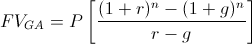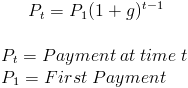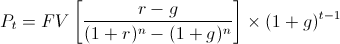# Growing Annuity Payment - FV

Growing Annuity Payment Calculator (FV) (Click Here or Scroll Down)The growing annuity payment formula using future value is used to calculate the first cash flow or payment of a series of cash flows that grow at a proportionate rate. A growing annuity may sometimes be referred to as an increasing annuity.

A few important things to note is that the growing annuity payment formula using future value shown calculates only the initial payment or cash flow. Each following cash flow from the first must be calculated separately. This is discussed further in the latter sections.

Another important issue to consider is that the formula used here is specifically used when the future value of the growing annuity is known. Determining whether to use the growing annuity payment formula for future value or to use the growing annuity payment formula for present value depends on if the balance of the annuity is increasing or decreasing. This is explained more in the following section.

## Use of the Growing Annuity Payment Formula using Future Value

To provide an example to better show an increasing vs. decreasing balance, suppose that an individual is wanting to calculate how much he can receive per year of his \$50,000 balance in his interest account in order for it to last 5 years. This individual would like for his cash flows to increase 5% per year to account for the relative purchasing power of the currency in his life. This example would use the present value version as the current balance in his account, \$50,000, is the present value of the future cash flows. The balance would be decreasing.

However, another example would be an individual who would like to calculate how much she would have to save per year, each annual deposit growing 10% per year, in order for her balance in an interest account to be \$50,000 in 5 years. The balance would be increasing in this scenario and the \$50,000 would be the future value. The growing annuity payment formula using future value would be used in this situation.

## How is the Growing Annuity Payment Formula using Future Value Derived?

The growing annuity payment formula using future value can be found by first looking at the future value of a growing annuity formula, which is:Notice that the initial payment, P, is the variable that is needed. Since the intent is to solve for P, the formula directly above can be rearranged as:From here, the numerator can be multiplied by the reciprocal of the denominator in order to arrive at the formula shown at the top of the page.

## Calculating a Future Payment for a Growing Annuity

As previously stated, the growing annuity payment formula using future value shown at the top of the page is specific to the first payment only. Because the payments grow at a proportionate rate, each individual cash flow will be different. A future payment can be calculated by the following formulaSince the cash flows grow at a proportionate rate, the growth rate can be used to find a future payment.

## Alternative Growing Annuity Payment Formula Using Future Value

The growing annuity payment formula using future value at the top of the page and the formula for calculating a future payment in the preceding section can be combined in order to calculate the payment for a particular period, denoted as time t:New to Finance?